##Feature Articles: Communication Science that Connects Information and Humans

# Fast Physical Random Number Generation Using Semiconductor Laser Chaos

## Abstract

We review recent developments in research on fast physical random number generation using a random phenomenon in semiconductor lasers. Random numbers are widely used in information security technology, and there is a need for high-quality, i.e., unpredictable and uniformly distributed, random numbers.

## 1. Introduction

A number (or a sequence of numbers) generated with no apparent rule is called a random number (random numbers). Random numbers are widely used for various purposes. Familiar examples are the number showing on a thrown dice or selected in roulette for gaming and a winning lottery number. Random numbers are also often used for computer simulation in both science and technology. In addition, the random number is an essential component of security technologies such as cryptographic systems and authentication systems.

There are two fundamental properties that should be possessed by random numbers. One is statistical uniformity, which is the property that the values of random numbers are uniformly distributed over their range. The other is unpredictability, which is the property that there is no means of fully or partially predicting the next value in a random number sequence. In particular, for security purposes, a random number must have unpredictability because this ensures a high security level. Therefore, it is crucial to develop a method of generating random sequences with these properties.

Some security technologies require a large quantity of random numbers in their operation. Typical examples are secret sharing schemes, which are used for securely storing data in storage devices by encrypting and dividing it, and quantum key distribution, which is expected to achieve the ultimate security. Therefore, there is a need for methods capable of generating random numbers at a high generation rate.

This article deals with a random number generation technique based on a random physical phenomenon, focusing on the method using fast random oscillation exhibited by semiconductor lasers. This method has the potential to generate high-quality unpredictable random numbers and achieve a high generation rate, and there has been great interest in it recently. We review recent developments in the research of fast physical random number generation using semiconductor lasers. More detailed technical reviews are given in Refs.  and .

## 2. Types of random number generation methods

Roughly speaking, there are two types of random number generation methods.

The first method produces pseudorandom numbers. It is a computational method. Given an initial number a0 called a seed, the method computes the next value a1 from a0 via a prescribed mathematical formula and then computes a2 from a1, and so on. The sequence a0, a1, a2 ... seems to be a random sequence, provided that an appropriate formula is used. Because of its computational nature, it is in principle possible to predict the sequence if both the formula and the seed become known. Pseudorandom number generators for security purposes are devised in such a manner that it will be difficult to determine the seed from the generated sequence. However, it is still impossible for pseudorandom number generators to achieve complete unpredictability.

The other method produces physical random numbers by utilizing randomness in physical phenomena. Typical and well known examples are dice and roulette. In addition, there are technologically more useful methods, which are based on the measurement of thermal noise in an electrical circuit or measurement of a quantum optical phenomenon. Compared with pseudorandom numbers, physical random numbers have the great advantage that can achieve complete unpredictability if an appropriate random physical phenomenon is used. However, the existing methods have the disadvantage of a low generation rate.

From the viewpoint of security applications, it is important to develop a random number generator that is guaranteed to have unpredictability and can achieve a high generation rate.

## 3. Chaos in semiconductor lasers

In a variety of systems in nature and technology, the time evolution of the system is described by a rule such that its future state is uniquely determined from its present and past states. Such a rule is called a deterministic rule. It has been known that systems governed by deterministic rules often exhibit irregular and very complex behaviors. Such a phenomenon with irregular and complex behavior is called chaos. The remarkable feature of chaos is a sensitive dependence on the initial state; i.e., when there are two identical systems with slightly different states at the initial, the difference rapidly becomes large with time.

Nowadays, it is recognized that chaos is not a rare anomaly but a ubiquitous phenomenon. Chaos can be easily observed in semiconductor lasers. In a semiconductor laser with optical feedback, the laser’s output light is reflected by a mirror and injected back into the laser (Fig. 1). An ordinary semiconductor laser, which has no feedback, emits light with constant intensity; by contrast, a semiconductor laser with optical feedback is intrinsically unstable, and the intensity of its output light exhibits irregular and complex oscillation. An example of the waveform of light output from a semiconductor laser with optical feedback is shown in Fig. 2. This experimental result clearly shows that chaos, which is characterized by irregular and complex oscillation, occurs in a semiconductor laser with optical feedback.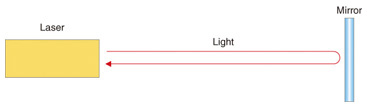Fig. 1. Laser with optical feedback.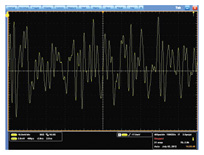Fig. 2. Intensity of output light of laser with optical feedback.

The chaos in a semiconductor laser with optical feedback has been extensively studied over the last three decades from the viewpoint of basic research. This subject has been being studied in collaborative research between Dr. Uchida’s group at Saitama University and NTT Communication Science Laboratories. Recently, we focused attention on the fast irregular oscillation in a semiconductor laser with optical feedback, which has broad bandwidth of the order of several gigahertz, and we proposed its application to fast random number generation.

## 4. Random number generation using semiconductor lasers

A simple method for obtaining binary random bits from the analog output waveform of a semiconductor laser with optical feedback is to periodically sample the waveform and digitize the sampled values by comparing them with a prescribed threshold: if the sampled value is smaller than the threshold, it is digitized to 0, otherwise to 1. Our research group has been developing a scheme for fast random number generation based on this simple method.

### 4.1 System configuration

The configuration of our experimental laser random number generator system, which has been developed in research collaboration with Dr. Uchida’s group (at Takushoku University at that time) is shown in Fig. 3. Although it is in principle possible to generate random bits with only one laser, our system in Fig. 3 consists of two independent lasers. This system configuration was devised to remove effects due to the weak periodicity of a single laser output. The use of two lasers improves the quality of random bits generated at a high generation rate.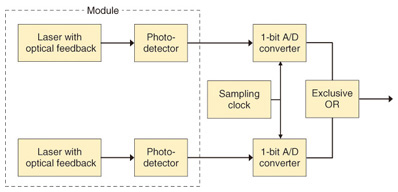Fig. 3. Configuration of random number generator using laser with optical feedback.

In Fig. 3, each semiconductor laser with optical feedback emits output light, and the output light intensity is measured by a photodetector. Each measured intensity value is converted into a binary value by an analog-to-digital (A/D) converter. The final output bit is obtained by applying an exclusive OR operation between the two binary values. Using this experimental system, we succeeded in achieving a random bit generation rate of 1.7 Gbit/s in November 2008; this was a world record for physical random bit generation rate . Since this pioneering work, fast random bit generation using a chaotic laser has become an active research area studied at many institutes around the world.

### 4.2 Challenges

The most advantageous feature of using a semiconductor laser in random bit generation is its potential high-speed performance, which can be much faster than the rate achievable with electrical circuits. It is of course important to increase the random bit generation rate by fully utilizing the high-speed performance. On the other hand, from the viewpoint of security applications, it is essential to guarantee the unpredictability of generated random bits so that users can use the bits without anxiety. In addition, from a practical viewpoint, it is necessary to make a system that is compact. We have been working on these important issues.

Our first experimental system developed in 2008 was composed of a number of optical components, and its size was about 1 m × 1 m, which is not small enough for practical use. Therefore, we applied optical integrated circuit technology developed by NTT Photonics Laboratories to our system and succeeded in drastically reducing the system size in 2010. The optical integrated circuit, which is about 10 mm × 0.3 mm, is shown in Fig. 4. It incorporates a semiconductor laser with optical feedback. A distributed feedback semiconductor laser (DFB) emits light, which propagates along the waveguide (black line in Fig. 4) and is reflected by a mirror placed at the right end to be reinjected into the DFB laser. This integrated circuit also has a photodetector at the left end.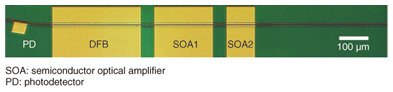Fig. 4. Optical integrated circuit of laser with optical feedback.

A module containing two of the optical integrated circuits shown in Fig. 4 is shown in Fig. 5. This module can perform the same function as the components enclosed by dashed lines in Fig. 3. It generates two irregularly fluctuating signals, which are the intensities of light output from the two built-in optical integrated circuits. Random bits can be obtained by digitizing these signals and applying an exclusive OR operation, as shown in Fig. 3. We have shown that it is possible to generate random bits at the rate 2.08 Gbit/s by using this module .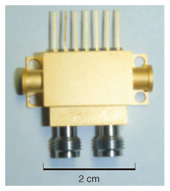Fig. 5. Module with two built-in optical integrated circuits.

The other important issue is the unpredictability of generated random bits. We have developed a theory for ensuring unpredictability , which we outline below. It is known that there is some unavoidable small quantum noise called spontaneous emission noise in any laser. This spontaneous emission noise is the origin of the unpredictability. Because of this noise, the state of a laser at any given moment cannot be determined uniquely, so it is necessary to consider that the laser state is distributed according to a certain probability distribution. The small uncertainty arising from this probability distribution rapidly becomes large within a short time because of the dynamical instability due to the chaos in a laser with optical feedback. This enlarged uncertainty leads to the uncertainty in the output light intensity waveform and hence to that in the random bits. Our computer simulation indicates that even if you know the state of a laser with optical feedback exactly at a certain moment, you cannot predict the intensity of its output light after 1 ns at all .

An experimental result confirming the rapid increase in uncertainty in a single semiconductor laser with optical feedback is shown in Fig. 6. In this experiment, we used the optical integrated circuit in Fig. 4, reset the system to the same initial state for each trial, and allowed the system to evolve. Figure 6 shows the time evolution of the output light intensity obtained for three trials. In the initial stage, the three curves are close to each other. By contrast, after 0.5 ns, the three curves are significantly different. This demonstrates that a small initial uncertainty rapidly increases to the macroscopic output level within only 0.5 ns.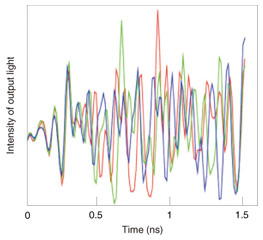Fig. 6. Experimental result for amplification of uncertainty.

## 5. Future perspective

The goal of our research is to establish the theoretical and experimental basis for physical random number generation technology using chaos in lasers. For this purpose, important issues are to generalize the theory guaranteeing the unpredictability of random bits and to develop a technique for experimentally confirming the theory. We are conducting research on these issues.

## References

 A. Uchida, “Review on ultra-fast physical random number generators based on optical random phenomena,” The Review of Laser Engineering, Vol. 39, No. 7, pp. 508–514, 2011 (in Japanese). T. Harayama, S. Sunada, and K. Tsuzuki, “Optical integrated circuits for laser chaos and fast physical random number generation,” The Review of Laser Engineering, Vol. 39, No. 7, pp. 515–519, 2011 (in Japanese). A. Uchida, K. Amano, M. Inoue, K. Hirano, S. Naito, H. Someya, I. Oowada, T. Karashige, M. Shiki, S. Yoshimori, K. Yoshimura, and P. Davis, “Fast physical bit generation with chaotic semiconductor lasers,” Nature Photonics, Vol. 2, No. 12, pp. 728–732, 2008. T. Harayama, S. Sunada, K. Yoshimura, P. Davis, K. Tsuzuki, and A. Uchida, “Fast nondeterministic random-bit generation using on-chip chaos lasers,” Physical Review A, Vol. 83, No. 3, 031803, 2011.Kazuyuki Yoshimura Distinguished Researcher, Media Information Laboratory, NTT Communication Science Laboratories. He received the B.E. degree in engineering physics from Kyoto University and the M.E. degree in aeronautics and astronautics from the University of Tokyo in 1992 and 1994, respectively. He received the Ph.D. degree in applied mathematics and physics from Kyoto University in 1997. He joined NTT in 1997. He was a visiting scholar at the University of California, San Diego, USA, during 2001–2002. His research interests are in nonlinear dynamics and its applications to communications. He is a member of the Physical Society of Japan, the Japan Society for Industrial and Applied Mathematics, the Institute of Electronics, Information and Communication Engineers (IEICE), and the Japan Society for Aeronautical and Space Sciences. Susumu Shinohara Research Specialist, Media Information Laboratory, NTT Communication Science Laboratories. He received the Ph.D. degree in physics from Waseda University, Tokyo, in 1999. He joined NTT as a research specialist in 2012 after working at Waseda University, Ritsumeikan University, Advanced Telecommunications Research Institute International, and Max Planck Institute for the Physics of Complex Systems. His research interests are in nonlinear physics, classical and quantum chaos, and their applications. He is a member of the Physical Society of Japan (JPS). Kenichi Arai Senior Research Scientist, Media Information Laboratory, NTT Communication Science Laboratories. He received the B.S. and M.S. degrees both in pure and applied physics from Waseda University, Tokyo, in 1991 and 1993, respectively, and the Ph.D. degree from Waseda University in 2003. He joined NTT Communication Science Laboratories in 1993. His research interests are in nonlinear dynamics, stochastic systems, neural networks, and complex networks. He is a member of IEICE and JPS.

↑ TOP